# GMAT Math : Calculating the length of a line with distance formula

## Example Questions

### Example Question #11 : Lines

A line segement on the coordinate plane has endpoints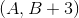and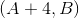. Which of the following expressions is equal to the length of the segment?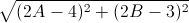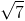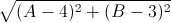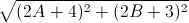Explanation:

Apply the distance formula, setting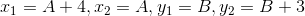: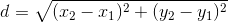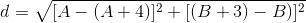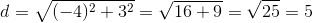### Example Question #12 : Lines

What is distance between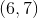and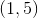?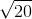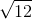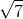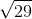Explanation: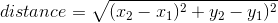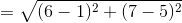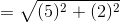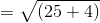### Example Question #851 : Gmat Quantitative Reasoning

What is the distance between the points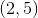and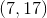?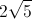Explanation:

Let's plug our coordinates into the distance formula.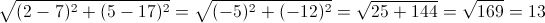### Example Question #14 : Lines

What is the distance between the points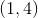and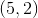?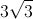Explanation:

We need to use the distance formula to calculate the distance between these two points.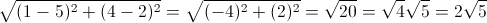### Example Question #15 : Lines

Consider segment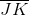which passes through the points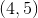and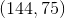.

Find the length of segment.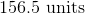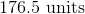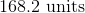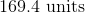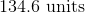Explanation:

This question requires careful application of distance formula, which is really a modified form of Pythagorean theorem.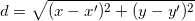Plug in everthing and solve: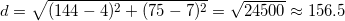### Example Question #16 : Lines

What is the length of a line segment that starts at the point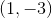and ends at the point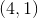?Explanation:

Using the distance formula for the length of a line between two points, we can plug in the given values and determine the length of the line segment by calculating the distance between the two points: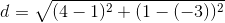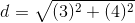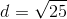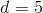Tired of practice problems?

Try live online GMAT prep today.